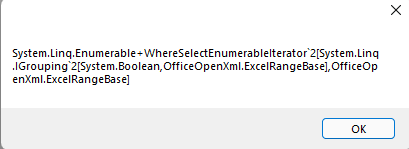Post

# How to get the last non-empty row number using EPPlus & c#?

Dec 19 2021 6:01 AMHow can I programtically find out the last non-empty row of a particular column (say column 1) using EPPlus & c#?

For testing I've create a test.xlsx file (shown below)

 ID Name Address Status 002 John USA

So, in this case the last non-empty row of column 1 should be 2. I've tried the below code but it shows me 10 instead of 2 (I think since I've formatted the cells till cell D10 like added some border?)

``````var myFile = new ExcelPackage(new FileInfo(@"test.xlsx"));

Excelsheet sheet = myFile.Workbook.sheets;

MessageBox.Show(sheet.Dimension.End.Row.ToString());``````

So I tried

``````var lastRowOfCol1 = sheet.Cells.Where(c=>c.Start.Column == 1 && c.End.Column == 1).GroupBy(x=>x.Value.ToString() !="").Select(p=>p.Last());

MessageBox.Show(lastRowOfCol1.ToString());``````

Now I'm gettingCan anyone help me on this?